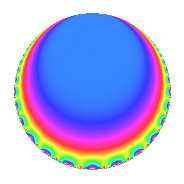# Properties

 Label 2016.1.l.aLevel 2016 Weight 1 Character orbit 2016.l Self dual Yes Analytic conductor 1.006 Analytic rank 0 Dimension 1 Projective image $$D_{2}$$ CM/RM disc. -7, -56, 8 Inner twists 4

# Related objects

## Newspace parameters

 Level: $$N$$ = $$2016 = 2^{5} \cdot 3^{2} \cdot 7$$ Weight: $$k$$ = $$1$$ Character orbit: $$[\chi]$$ = 2016.l (of order $$2$$ and degree $$1$$)

## Newform invariants

 Self dual: Yes Analytic conductor: $$1.00611506547$$ Analytic rank: $$0$$ Dimension: $$1$$ Coefficient field: $$\mathbb{Q}$$ Coefficient ring: $$\mathbb{Z}$$ Coefficient ring index: $$1$$ Projective image $$D_{2}$$ Projective field Galois closure of $$\Q(\sqrt{2}, \sqrt{-7})$$ Artin image size $$8$$ Artin image $D_4$ Artin field Galois closure of 4.0.14112.1

## $q$-expansion

 $$f(q)$$ $$=$$ $$q$$ $$\mathstrut +\mathstrut q^{7}$$ $$\mathstrut +\mathstrut O(q^{10})$$ $$q$$ $$\mathstrut +\mathstrut q^{7}$$ $$\mathstrut +\mathstrut 2q^{23}$$ $$\mathstrut -\mathstrut q^{25}$$ $$\mathstrut +\mathstrut q^{49}$$ $$\mathstrut -\mathstrut 2q^{71}$$ $$\mathstrut +\mathstrut 2q^{79}$$ $$\mathstrut +\mathstrut O(q^{100})$$

## Character Values

We give the values of $$\chi$$ on generators for $$\left(\mathbb{Z}/2016\mathbb{Z}\right)^\times$$.

 $$n$$ $$127$$ $$577$$ $$1765$$ $$1793$$ $$\chi(n)$$ $$1$$ $$-1$$ $$-1$$ $$1$$

## Embeddings

For each embedding $$\iota_m$$ of the coefficient field, the values $$\iota_m(a_n)$$ are shown below.

For more information on an embedded modular form you can click on its label.

Label $$\iota_m(\nu)$$ $$a_{2}$$ $$a_{3}$$ $$a_{4}$$ $$a_{5}$$ $$a_{6}$$ $$a_{7}$$ $$a_{8}$$ $$a_{9}$$ $$a_{10}$$
433.1
 0
0 0 0 0 0 1.00000 0 0 0
 $$n$$: e.g. 2-40 or 990-1000 Significant digits: Format: Complex embeddings Normalized embeddings Satake parameters Satake angles

## Inner twists

Char. orbit Parity Mult. Self Twist Proved
1.a Even 1 trivial yes
7.b Odd 1 CM by $$\Q(\sqrt{-7})$$ yes
8.b Even 1 RM by $$\Q(\sqrt{2})$$ yes
56.h Odd 1 CM by $$\Q(\sqrt{-14})$$ yes

## Hecke kernels

This newform can be constructed as the kernel of the linear operator $$T_{11}$$ acting on $$S_{1}^{\mathrm{new}}(2016, [\chi])$$.